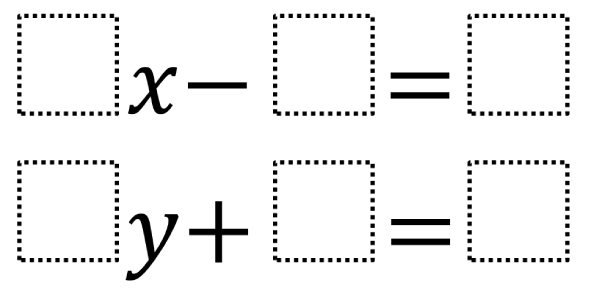# Two Step Equations Quiz: Test!

10 Questions | Total Attempts: 8047SettingsA two-step equation is an algebraic equation. You will need to perform two steps to solve the equation. This practice quiz has been made to test your calculation skills on solving two-step equations. All the best!

• 1.
6 = a/4 + 2
• A.

A = 24

• B.

A = 4

• C.

A = -16

• D.

A = 16

• 2.
9x − 7 = −7
• A.

X = 0

• B.

X = 1

• C.

X = 49

• D.

X =-63

• 3.
0 = 4 + n/5
• A.

N = -20

• B.

N = -1

• C.

N= 20

• D.

N = 0

• 4.
−1 = 5 + x/6
• A.

X = 4

• B.

X = -36

• C.

X = -6

• D.

X = 30

• 5.
−9x + 1 = −80
• A.

X = -81

• B.

X = -10

• C.

X = 9

• D.

X = 0

• 6.
−10 = −10 + 7m
• A.

M = 1

• B.

M = 0

• C.

M = -70

• D.

M = -1

• 7.
144 = −12x - 60
• A.

X = -12

• B.

X = 5

• C.

X = - 5

• D.

X = -17

• 8.
8n + 7 = 31
• A.

N = -2

• B.

N = 4

• C.

N = 1

• D.

N = 3

• 9.
M/9 − 1 = −2
• A.

M = -1/3

• B.

M = 27

• C.

M = 9

• D.

M = -9

• 10.
Solve the equation. 6x - 7 = 35
• A.

X = -7

• B.

X = 0

• C.

X = 5

• D.

X = 7

Related TopicsBack to top